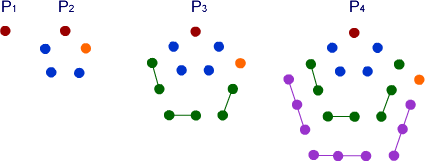#### You may also like### Diophantine N-tuples

Can you explain why a sequence of operations always gives you perfect squares?### DOTS Division

Take any pair of two digit numbers x=ab and y=cd where, without loss of generality, ab > cd . Form two 4 digit numbers r=abcd and s=cdab and calculate: {r^2 - s^2} /{x^2 - y^2}.### Sixational

The nth term of a sequence is given by the formula n^3 + 11n . Find the first four terms of the sequence given by this formula and the first term of the sequence which is bigger than one million. Prove that all terms of the sequence are divisible by 6.

# Triangles Within Pentagons

##### Age 14 to 16Challenge LevelThe diagram above shows the pentagon growing in a systematic way to produce the pentagonal numbers. The equations below describe this growth:
$$\begin{eqnarray} P_2 &=& P_1 + 4 \\ &=&1+4 \\ &=&1 + 3 \times 1 + 1\\ P_3&=& P_2 + 7 \\ &=& 1+4+7 \\ &=& 1 + (3 \times 1 + 1) + (3 \times 2 + 1) \\ P_4 &=& P_3 + 10 \\ &=& 1 + (3 \times 1 + 1) + (3 \times 2 + 1) + (3 \times 3 + 1) \\ \end{eqnarray}$$

Can you find a general rule for $P_n$?

By writing a formula for the nth triangular number $T_n$, show that all pentagonal numbers are one third of a triangular number and prove that the triangular numbers involved are all of the form $T_{3n-1}$